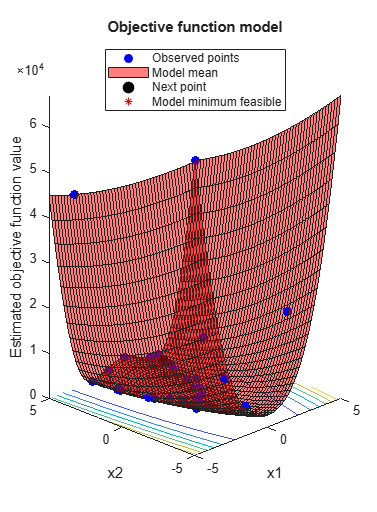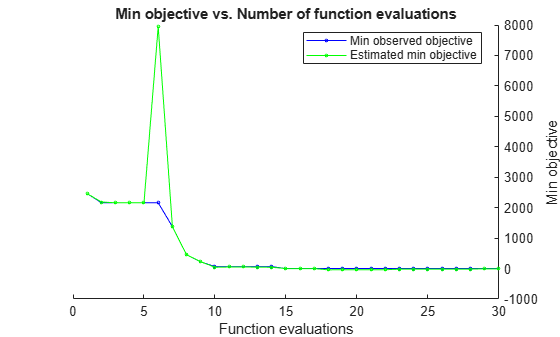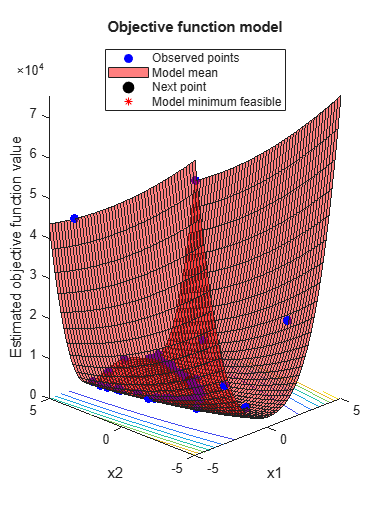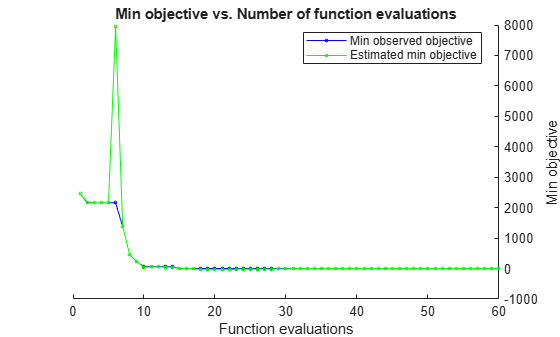resume

Resume a Bayesian optimization

Description

example

newresults = resume(results,Name,Value) resumes the optimization that produced results with additional options specified by one or more Name,Value pair arguments.

Examples

collapse all

This example shows how to resume a Bayesian optimization. The optimization is for a deterministic function known as Rosenbrock's function, which is a well-known test case for nonlinear optimization. The function has a global minimum value of 0 at the point [1,1].

Create two real variables bounded by -5 and 5.

x1 = optimizableVariable('x1',[-5,5]);
x2 = optimizableVariable('x2',[-5,5]);
vars = [x1,x2];

Create the objective function.

function f = rosenbrocks(x)

f = 100*(x.x2 - x.x1^2)^2 + (1 - x.x1)^2;

fun = @rosenbrocks;

For reproducibility, set the random seed, and set the acquisition function to 'expected-improvement-plus' in the optimization.

rng default
results = bayesopt(fun,vars,'Verbose',0,...
'AcquisitionFunctionName','expected-improvement-plus');View the best point found and the best modeled objective.

results.XAtMinObjective
results.MinEstimatedObjective
ans =

1x2 table

x1        x2
______    ______

1.7902    3.2287

ans =

-9.1194

The best point is somewhat close to the optimum, but the function model is inaccurate. Resume the optimization for 30 more points (a total of 60 points), this time telling the optimizer that the objective function is deterministic.

newresults = resume(results,'IsObjectiveDeterministic',true,'MaxObjectiveEvaluations',30);
newresults.XAtMinObjective
newresults.MinEstimatedObjective
ans =

1x2 table

x1         x2
_______    _______

0.95093    0.90364

ans =

-0.0109The objective function model is much closer to the true function this time. The best point is closer to the true optimum.

Input Arguments

collapse all

Bayesian optimization results, specified as a BayesianOptimization object.

Name-Value Arguments

Specify optional comma-separated pairs of Name,Value arguments. Name is the argument name and Value is the corresponding value. Name must appear inside quotes. You can specify several name and value pair arguments in any order as Name1,Value1,...,NameN,ValueN.

Example: resume(results,'MaxObjectiveEvaluations',60)

You can use any name-value pair accepted by bayesopt except for those beginning with Initial. See the bayesopt Input Arguments.

Note

The MaxObjectiveEvaluations and MaxTime name-value pairs mean additional time or evaluations, above the numbers stored in results. So, for example, the default number of evaluations is 30 in addition to the original specification.

Additionally, you can use the following name-value pair.

Modify variable, specified as an OptimizableVariable object.

You can change only the following properties of a variable in an optimization.

• Range of real or integer variables. For example,

xvar = optimizableVariable('x',[-10,10]);
% Modify the range:
xvar.Range = [1,5];
• Type between 'integer' and 'real'. For example,

xvar.Type = 'integer';
• Transform of real or integer variables between 'log' and 'none'. For example,

xvar.Transform = 'log';

Output Arguments

collapse all

Optimization results, returned as a BayesianOptimization object.